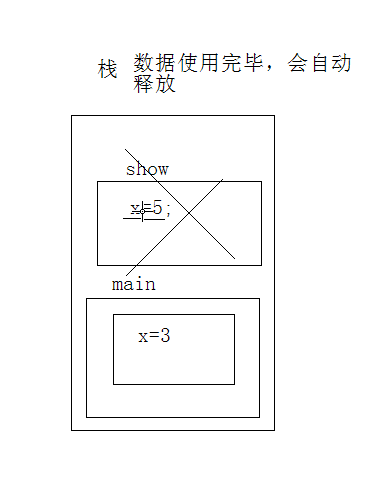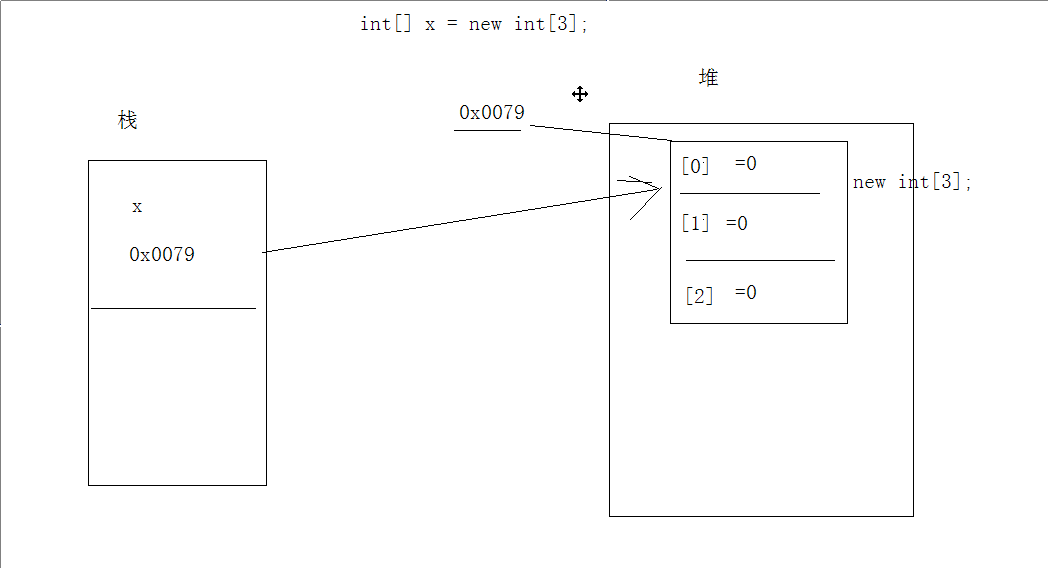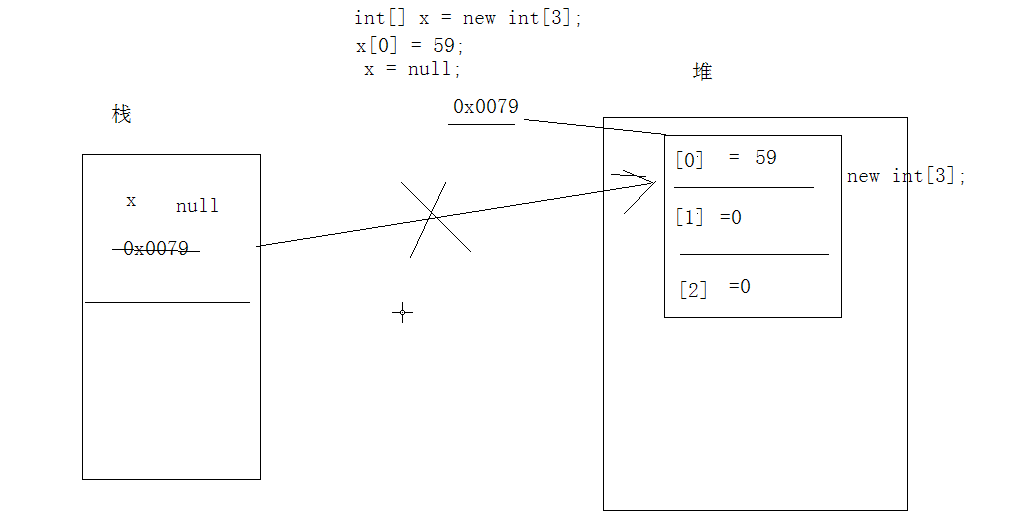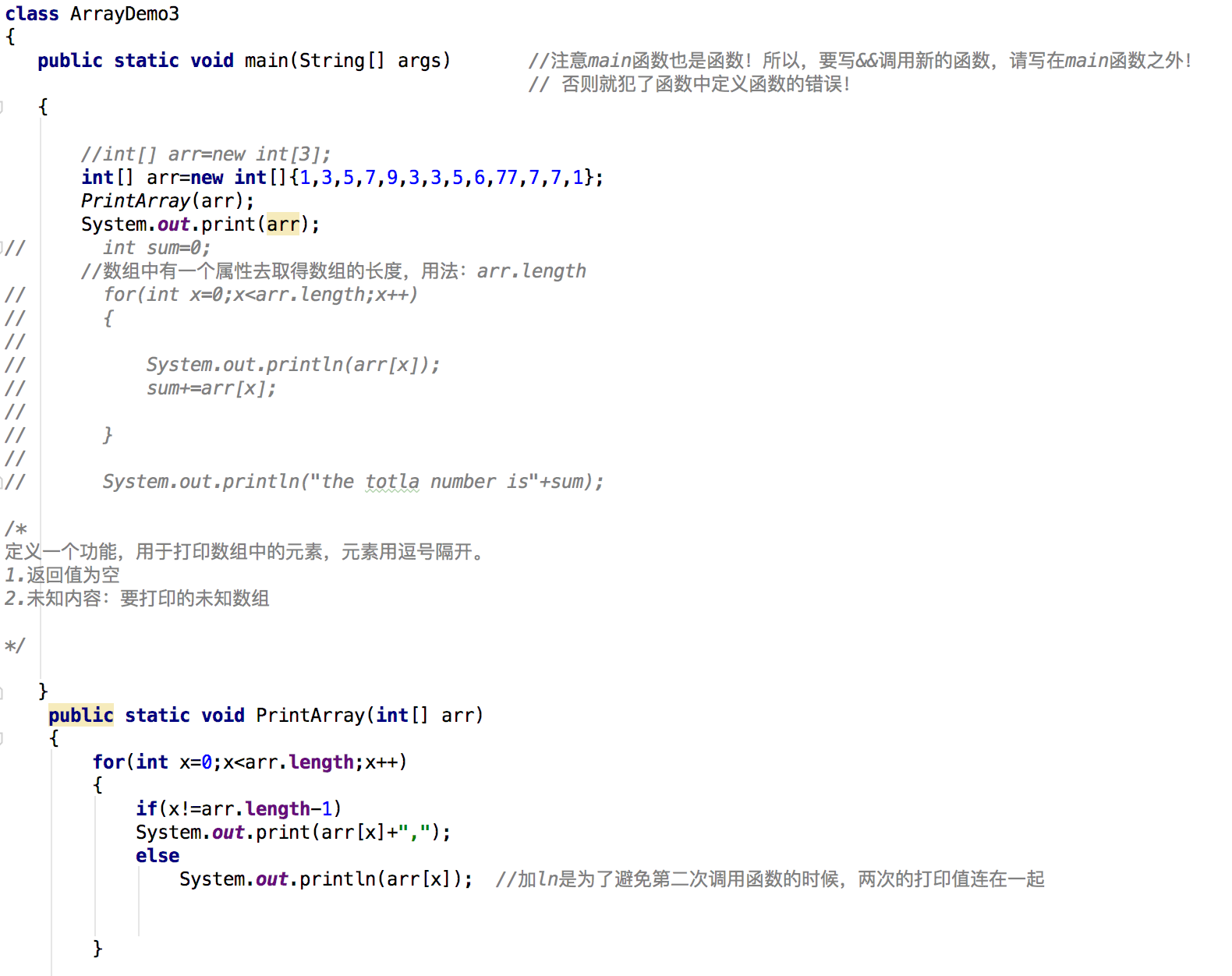# D0317数组（概述-内存结构）&&D401~03数组常见操作2.8.1 数组的定义

//想定义一个存储3个整数的容器
int[] x=new int; //x的数据类型：数组；加[]就是为了说明x是数组类型
//打印数组中角标为0的元素
System.out.println(x);

int[] arr=new int[]{3,5,1,7};
int[] arr={3,5,1,7};

2.8.2内存结构
java程序在运行时，需要在内存中分配空间。为了提高运算效率，有对空间进行了不同领域的划分，因为每一片区域都有特定的处理数据方式和内存管理方式。

E.g.E.g.java语言承仿于C++,优化了C++语言的特性，尤其是在内存这一块。当内存中的实体越来越多，C++是需要程序员手动调用一个功能，如果程序员忘记了，程序越长，运行越慢甚至最后会死机。而java是一旦发现一个实体没人引用就把这个实体当做垃圾，不定时的启动垃圾启动机制来清除。
E.g.
int x[]=new int;
int y[]=x; //两个引用指向同一个实体
y=89;
x=null;D0401 数组（静态初始化-常见问题）

int[] arr=new int; //中括号和数据类型或者数据名称放在一起，两种写法都可以
int arr[]=new int;

int arr[]=new int[]{3,1,5,7,6};//数据明确的情况下可以这么写

int[] arr=new int;
System.out.print(arr); //输出显示为0.（m默认初始值）
System.out.print(arr); //没有角标为3的数值，但是编译不报错。编译只检查语法错误。在运行的时候才会在堆内存中去开辟一个数组空间，并分配0、1、2这三个角标，去找的时候发现角标不存在，报错”ArrayIndexOutofBoundsException:3”
//类似报错”ArrayIndexOutofBoundsException:3”的情况，一般是操作数组时，操作到了数组中不存在的角标

int[] arr=new int;
arr=null;
System.out.print(arr); //NullPointerException:空指针异常。表示arr这个引用已经不再指向任何的数组实体了，值为null

D0402 数组（常见操作-遍历）[表示数组类型的引用
I表示存放int类型的数据
@后面跟的d041cf表示内存中的地址（十六进制的数，由哈希算法算出来的哈希值）
D0403 数组（常见操作）

D0403 数组（常见操作-获取最值）
class ArrayDemo3
{
public static void main(String[] args)
{
int[] arr=new int[]{5,1,7,4,3,9};
// GetMaxValue(arr);//这么写不会有任何的显示!
// System.out.print(GetMaxValue(arr)); //此时才会打印输出9
// int max=GetMaxValue(arr);
int min=GetMinIndex(arr);
System.out.println(“min=”+min);

}

/*

1.获取最值必须要进行比较。每一次比较都会有一个较大的值，因为该值不确定，通过一个变量进行临储。
2.让数组中的元素都和这个变量中的值进行比较，如果大于变量中的值，就用该变量记录较大的值。
3.当所有的元素都比较完成，该变量中存储的就是数组中最大的值
*/

`````` public static int GetMaxValue(int[ ] arr)
{
int max=arr;
for(int x=1;x<arr.length;x++)
{

if(arr[x]>max)
max=arr[x];

}

return max;
}
``````

/*获取最大值的一种方式：

*/

``````public static int GetMaxValue1(int[ ] arr)
{
int max=0;
for(int x=1;x<arr.length;x++)
{

if(arr[x]>max)
max=x;

}

return max;
}
``````

/*

*/

``````public static int GetMinIndex(int[ ] arr)
{
int min=0;
for(int x=1;x<arr.length;x++)
{

if(arr[x]<arr[min])
min=x;

}

return arr[min];
``````

}

/获取double类型数组的最大值，因为功能一致，所以定义形同函数名称，以重载形式存在/
public static double GetMaxValue(double[ ] arr)
{
int min = 0;
for (int x = 1; x < arr.length; x++)
{

``````    if (arr[x] < arr[min])
min = x;

}

return arr[min];
``````

}
}

``````                    2018.5.31
``````